# Data Structure and algorithm interview questions in java

I have been posting data structure and algorithms interview questions on various topics such as Array, Queue, Stack, Binary tree, LinkedList, String, Number, ArrayList, etc. So I am consolidating a list of programs to create an index post. I will keep adding links to this post whenever I add new programs. These are frequently asked Data Structure and algorithm interview questions.

If you want to practice and improve data structure and algorithm programs, this post will be very helpful to you. I will recommend you to try it yourself first and then check the solution.

## Stack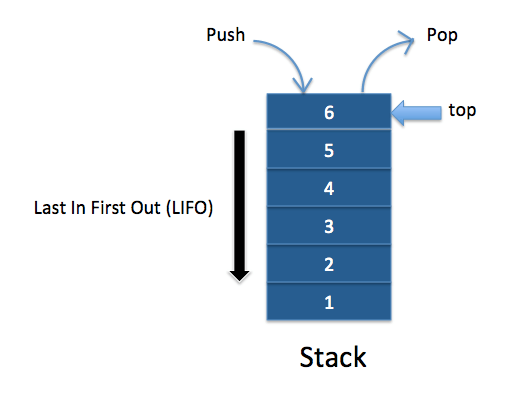### Question 1:  Implement a stack using array.

You need to implement Stack using array. You need to write push and pop methods to demonstrate Stack behavior( Last In First Out).
Solution : Java Program to implement stack using array.

### Question 2: Implement a stack using Linked List.

You need to implement Stack using Linked List. You need to write push and pop methods to demonstrate Stack behavior( Last In First Out).
Solution  Java Program to implement stack using Linked List

### Question 3:  Implement a stack using two queues.

You need to use two queues to implement stack behavior.You need to write push and pop methods to demonstrate Stack behavior( Last In First Out).
Solution : Java Program to implement stack using two queues

### Question 4 : Sort an stack using another stack

You need to sort an stack using another stack. You can use push and pop operation of stack to do so,
Solution : Sort a stack using another stack.

## Queue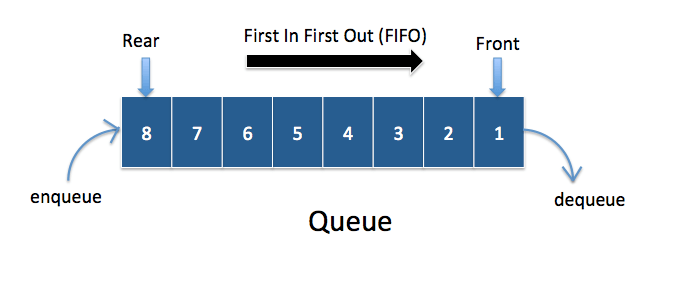### Question 5:  Implement Queue using Array in java.

You need to use array to implement queue.
Solution : Implement Queue using Array in java

### Question 6:  Implement a stack using two queues .

You need to use Linked list to implement queue.
Solution : Java Program to implement queue using linked list

### Question 7 : Implement singly linked list in java.

You need to implement singly linked list data structures.You need to write simple program to demonstrate insert , delete operations.

### Question 8: How to reverse linked list in java.

You need to write iterative and recursive solution to reverse linked list.
Solution : Java program to reverse linked list in java.

### Question 9: How to find middle element of linked list.

You need to write java program to find middle element of linked list in most optimize way.

### Question 10 : How to find nth element from end of linked list .

You need to write java program to find nth  element of linked list in most optimize way.
In question 6, Node 7 is 3rd from last of linked list.
Solution : How to find nth element from end of linked list.

### Question 11 : How to detect a loop in linked list. If linked list has loop, find the start node for the loop.

You need to write a java program to detect whether any loop exists in linked list and if loop exists , you need to find start node for the linked list.
Solution : How to detect loop in linked list.
How to find start node of loop in linked list.

### Question 12: How to check if linked list is palindrome or not?

A palindrome is a word, phrase, number, or other sequence of symbols or elements that reads the same forward or reversed. For example: 12121 is palindrome as it reads same forward or reversed. madam is also a palindrome . So we need write java programs to check if linked list is palindrome or not.
Solution : Java program to check if linked list is palindrome.

### Question 13 :  Find intersection of two linked lists?

Given two singly linked lists, find if two linked lists intersect. If they intersect, find intersection point.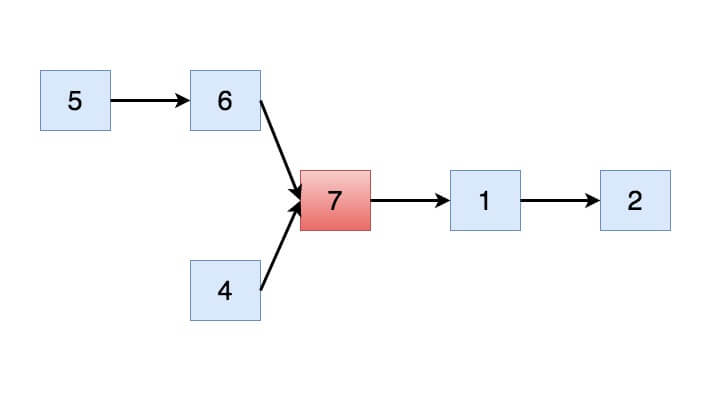Solution  : Intersection of two linked list

### Question 14 :  How to reverse a linked list in pairs?

You need to write a java program to reverse linked list in pairs.

Solution  : Java program to reverse linked list in pair.

### Question 15 :  Implement Doubly linked list in java?

You need to write a java program to implement doubly linked list in java.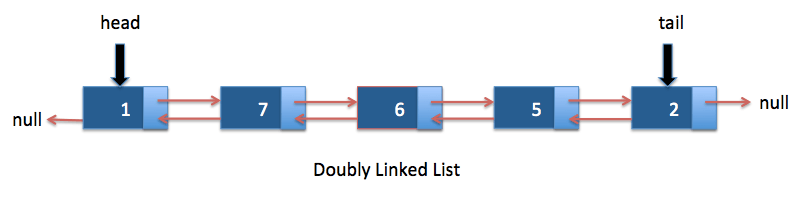Solution  : Doubly Linked List in java

## Array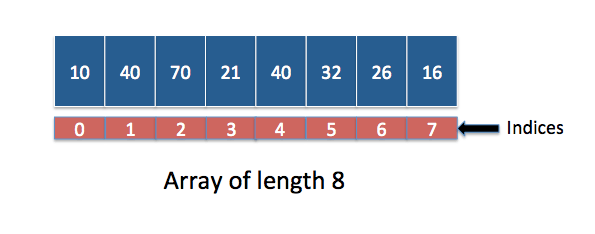### Question 16 : Write java Program to Find Smallest and Largest Element in an Array.

You are given an integer array containing 1 to n but one of the number from 1 to n in the array is missing. You need to provide an optimum solution to find the missing number. Number can not be repeated in the arry.
For example:

Solution : Java Program to Find Smallest and Largest Element in an Array

### Question 17 : Find missing number in the array.

You are given an integer array containing 1 to n but one of the number from 1 to n in the array is missing. You need to provide optimum solution to find the missing number. Number cannot be repeated in the arry.
For example:

Solution : Find missing number in the array.

### Question 18 : Search an element in rotated and sorted array.

You are given an sorted and rotated array as below:

If you note that array is sorted and rotated. You need to search an element in above array in o(log n) time complexity.
Solution : Search element in rotated and sorted array

### Question 19 : Find minimum element in a sorted and rotated array.

You are given an sorted and rotated array as below:

If you note that array is sorted and rotated. You need to i an element in above array in o(log n) time complexity.
Solution : Find minimum element in a sorted and rotated array

### Question 20: Find second largest number in an array

You are given an sorted and rotated array as below:
For example:

### Question 21 : Find the number occurring odd number of times in an array

You are given a array of integer. All numbers occur even number of times except one. You need to find the number which occurs odd number of time. You need to solve it with o(n) time complexity and o(1) space complexity.
For example:

### Question 22 : Find minimum number of platforms required for railway station

You are given arrival and departure time of trains reaching to a particular station. You need to find minimum number of platforms required to accommodate the trains at any point of time.

For example:

Please note that arrival time is in chronological order.

### Question 23 : Find a Pair Whose Sum is Closest to zero in Array

Given array of +ve and -ve integers ,we need to find a pair whose sum is closed to Zero in Array.
For example:

### Question 24 : Given a sorted array and a number x, find the pair in array whose sum is closest to x

Given a sorted array, we need to find a pair whose sum is closed to number X in Array.
For example:

### Question 25 : Find all pairs of elements from an array whose sum is equal to given number

Given a  array,we need to find all pairs whose sum is equal to number X.
For example:

For example:

### Question 27 : Separate odd and even numbers in an array

Given an array of integers, you need to segregate odd and even numbers in an array.
Please note: Order of elements can be changed.

For example:

For example:

### Question 29 : Find local minima in array

A local minima is less than its neighbours

For example:

### Question 30 : Sliding window maximum in java

Given an Array of integers and an Integer k, Find the maximum element of from all the contiguous subarrays of size K.

For example:

### Question 31 : Count number of occurrences (or frequency) of each element in a sorted array

Given a Sorted Array of integers containing duplicates. Find the frequency of every unique element present in the array.
Frequency is defined as the number of occurrence of any element in the array.

For example :

### Question 32 : Find subarrays with given sum in an array.

Given an Array of non negative Integers and a number. You need to print all the starting and ending indices of Subarrays having their sum equal to the given integer.
For example :

### Question 33 : Find peak element in the array.

Peak Element is the element of the array which is GREATER THAN / EQUAL TO its neighbours, that is, for an element at i th index, the neighbour elements at index i-1 & i+1 must be greater than equal to element at i th position.

### Question 34 : Find leaders in an array.

We need to print all the leaders present in the array. Element is the leader if it is greater than right side of elements.

For example:

### Question 35 : Count 1’s in sorted Binary Array.

Print number of 1’s in a given sorted Binary Array.
For example :

### Question 36 : Find first repeating element in an array of integers.

Find the first repeating element in array of integers.
For example :

### Question 37 : Check if Array Elements are Consecutive.

Given an array, we need to check if array contains consecutive elements.
For example :

### Question 38 : Permutations of array in java.

Given array of distinct integers, print all permutations of the array.
For example :

For example :

### Question 40 : Stock Buy Sell to Maximize Profit.

Given an array of integers representing stock price on single day, find max profit that can be earned by 1 transaction.
So you need to find pair (buyDay,sellDay) where buyDay < = sellDay and it should maximise the profit.
For example :

### Question 41 : Find maximum difference between two elements such that larger element appears after the smaller number.

Given array of integers, find Maximum difference between two elements such that larger element appears after the smaller number
For example :

### Question 42 : Search in a row wise and column wise sorted matrix.

Given row wise and column wise sorted matrix ,we need to search element with minimum time complexity.

### Question 43 : Largest sum contiguous subarray.

Largest sum contiguous subarray is the task of finding the contiguous subarray within a one-dimensional array of numbers which has the largest sum.
For example :

### Question 44 : Find the Contiguous Subarray with Sum to a Given Value in an array.

Given an array of positive integer and given value X, find Contiguous sub array whose sum is equal to X.
For example :

### Question 45 : Longest Common Prefix in an array of Strings in java.

Given an array of positive integer and given value X, find Contiguous sub array whose sum is equal to X.
For example :

### Question 46 : Find all subsets of set (power set) in java.

Given a set of distinct integers, arr, return all possible subsets (the power set).
For example :

## String

### Question 47 : How to reverse a String in java? Can you write a program without using any java inbuilt methods?

Solution: There are many ways to do it, some of them are:

• Using for loop
• Using recursion
• Using StringBuffer
Please refer to the solution at reverse a String in java

### Question 48 : Write a java program to check if two Strings are anagram in java?

Solution: Two string are anagrams if they have same characters but in different order. For example: Angel and Angle are anagrams
There are few ways to check if Strings are anagrams. Some of them are:

1. Using String methods
2. Using array.sort

### Question 49 : Write a program to check if String has all unique characters in java?

Solution: Here are some ways to check if String contains all unique characters

• By using HashSet
• Using indexOf and lastIndexOf methods of String
• By Using ascii value of characters.
Please refer to complete solution at check if String has all unique characters.

### Question 50 : How to check if one String is rotation of another String in java?

Solution: Let’s say you want to check whether str1 and str2 is rotation of one another or not.

1. Create a new String with str3= str1 + str1
2. Check if str3 contains str2 or not.
3. if str3 contains str2 then str2 is rotation of str1 else it is not
You can find complete solution at check if one String is rotation of another in java.

### Question 51 : How to find duplicate characters in String in java?

Solution:  Here is a solution to find duplicate characters in String.

1. Create a HashMap and character of String will be inserted as key and its count as value.
2. If Hashamap already contains char,increase its count by 1, else put char in HashMap.
3. If value of Char is more than 1, that means it is duplicate character in that String.

### Question 52 : Find first non repeated character in String in java?

Solution: There are may ways to find it.
Some of them are:

Please find complete solution at find first non repeated character in  a String.

### Question 53 : Find all substrings of String in java?

Solution: Java program to find all substrings of a String.
For example: If input is “abb”  then output should be “a”, “b”,”b”, “ab”, “bb”, “abb”
We will use String class’s subString method to find all subString.
Please refer to complete solution at find all subStrings of String.

### Question 54 : Find length of String without using any inbuilt method in java?

Solution: You can use try catch block for catching StringIndexOutOfBoundException and when this exception aries, you can simply return i(Index at which you will get the exception)
Please refer to complete solution at find length of String without inbuilt methods.

### Question 55 : Write a program to print all permutations of String in java?

Solution: Take out first character of String and insert into different places of permutations of remaining String recursively. Please find complete solution at how to find all permutations of String in java.

## Binary Tree

### Question 56 : How can you traverse binary tree?

There are three ways to traverse binary tree.

### Question 57 : Write an algorithm to do level order traversal of binary tree?

You need to write java program to do level order traversal of binary tree. You can use queue data structure to do level order traversal.

### Question 58 :  Write an algorithm to do spiral order traversal of binary tree?

You need to write java program to do spiral level order traversal of binary tree

### Question 59 : How can you print leaf nodes of binary tree?

You need to write java program to print all leaf nodes of binary tree.

Leaf nodes for above binary tree will be 5 , 30 , 55 ,70
Solution : Print leaf nodes of binary tree.

### Question 60 : How to count leaf nodes of binary tree.

You need to write java program to count leaf nodes of binary tree.
Count of Leaf nodes for binary tree used in Question 15 are 5.
Solution : Count leaf nodes of binary tree.

### Question 61 : How to print all paths from root to leaf in binary tree.

You need to write a program to print all paths from root to leaf.

### Question 62 : How to find level of node in binary tree

Given a node, you need to find level of a node. For example : Level of node will 3 for node 70 used in Question 14.
Solution: Find level of node in binary tree.

### Question 63 : How to find maximum element in binary tree.

You need to write a java program to find maximum element in binary tree.
Solution : Find maximum element in binary tree.

### Question 64 : How to find lowest common ancestor(LCA) in binary tree.

You need to write a program to find LCA in binary tree.

Solution: Program to find LCA in binary tree.

### Question 65 : How to do boundary traversal of binary tree.

Write a java program to do boundary traversal of binary tree as shown in below image.

Solution : Boundary traversal of binary tree.

### Question 66 : How to print vertical sum of binary tree?

You need to find sum of nodes which lies in same column.

Solution : How to print vertical sum of binary tree.

### Question 67 : Count subtrees with Sum equal to target in binary tree?

Given a Binary tree and an integer. You need to find the number of subtrees having the sum of all of its nodes equal to given Integer, that is, Target sum.

## Binary Search tree

### Question 68 : What is binary search tree?

Binary search tree is a special type of binary tree which have following properties.

• Nodes which are smaller than root will be in left subtree.
• Nodes which are greater than root will be right subtree.
• It should not have duplicate nodes
• Both left and right subtree also should be binary search tree.

### Question 71 :  How can you find minimum and maximum elements in binary search tree?

Solution : Leftmost and rightmost nodes of binary search tree are minimum and maximum nodes respectively

### Question 72 : How to find lowest common ancestor(LCA) in binary search tree.

You need to write a program to find LCA in binary search tree.

### Question 73 : Find inorder successor in a Binary search Tree

You need to write a program to find inorder successor in a Binary search tree.

## Graph

### Question 88 : Explain Dijkstra algorithm from source to all other vertices.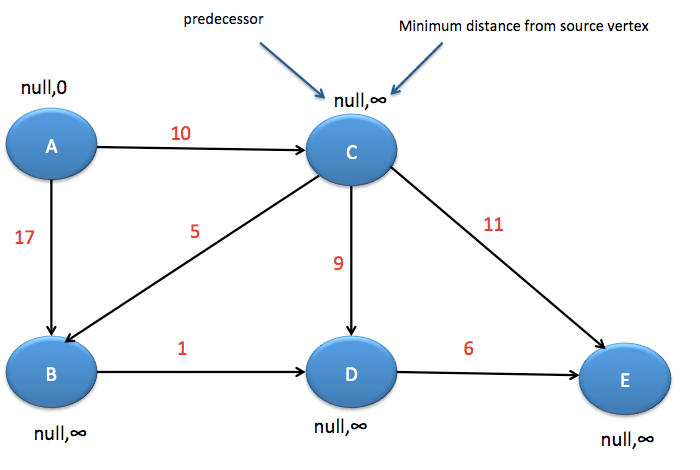### Question 89 : Explain Bellman Ford algorithm to find shortest distance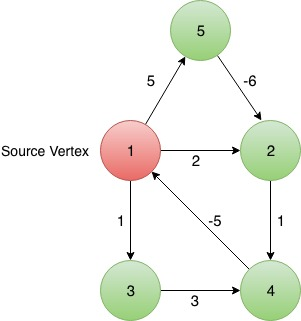### Question 90 : Explain Kruskal’s algorithm for finding minimum spanning tree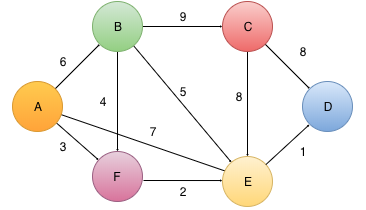## Dynamic Programming

### Question 91 : Given two String, find longest common substring.

Solution: Longest common substring in java.

### Question 92 : Given two Strings A and B. Find the length of the Longest Common Subsequence (LCS) of the given Strings.

Solution: Longest common subsequence in java

### Question 93 : Given a matrix, we need to count all paths from top left to bottom right of MxN matrix. You can either move down or right.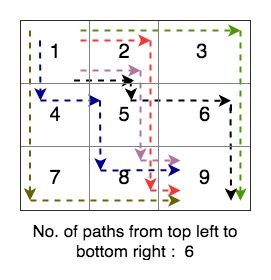Solution: Count all paths in matrix

### Question 94 : Edit Distance Problem in java

Given two strings string1 and string2, String1 is to be converted into String2 with the given operations available in the minimum number of steps. Using any one of the given operations contributes to the increment of steps by one.

Allowed Operations are :
(i) Remove : This operation allows the Removal any one character from String.
(ii) Insert : This operation allows the Insertion of one character at any spot in the String.
(iii) Replace : This operation allows the replacement of any one character in the string with
any other character.

Solution: Edit distance problem in java.

### Question 95: Coin change problem in java

Given an Amount to be paid and the currencies to pay with. There is infinite supply of every currency using combination of which, the given amount is to be paid. Print the number of ways by which the amount can be paid.

Solution: Coin change problem in java

## Miscellaneous

### Question 97 : What is an algorithm and how to calculate complexity of algorithms.

Solution : How to calculate Complexity of algorithm

### Question 98 : Implement trie data structure in java.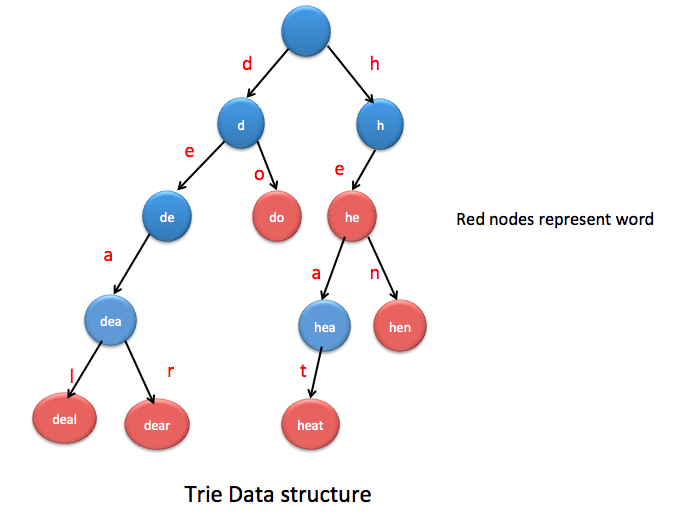Solution : Implement trie data structure in java.

### Question 99 : Count Factorial Trailing Zeroes in java.

Solution : Count Factorial Trailing Zeroes in java

### Question 102 : What is Memoization.

Solution :
Memoization ensures that method does not execute more than once for same inputs by storing the results in the data structure(Usually Hashtable or HashMap or Array).
Memoization example in java

This is all about questions on data structure and algorithm interview questions. Please do comment if you want to add any new questions to above list.
You may also like:

1.Navdeep December 10, 2016
2.premkumar July 1, 2017
3.pearl October 5, 2017
4.Raghav Sharma October 19, 2019
5.Suhani February 15, 2020